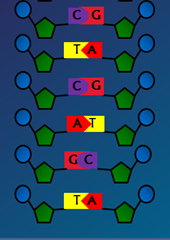# How to calculate the percentage of bases in a DNA strand using Chargaff’s rule? (Chargaff's Rule Questions)

According to Chargaff’s rule, the concentration of guanine approximately equals the concentration of cytosine.
In other words
The amount of purine = The amount of pyramidine in a given DNA molecule.

[A]+[G]=[C]+[T]

• Adenine (A) is paired to Thymine (T) by 2 hydrogen bonds
• Cytosine (C ) is paired to Guanine (G) by 3 hydrogen bonds
Genetics Problems:  Chargaff's Rule Questions
• Rule 1: The amount of Adenine ~equals the amount of Thymine

The amount of Guanine ~equals the amount of Cytosine

• The amount of purine = the amount of pyramidine

• Rule 2: The amount of A+T ≠ amount of G+C. This ratio varies among different organisms but same in different tissues of the same organism

Watch this simplified video that summarizes Chargaff''s Experiment, Rule and Practice problems1. A segment of DNA has 120 adenine and 120 cytosine bases. The total number of nucleotides present in the segment is
a) 60 (b)120 (c) 240 (d) 480
According to Chargaff rule,
• Adenine (A) is paired to Thymine (T)
• Cytosine (C ) is paired to Guanine (G)
Here  adenine residues =120, cytosine residues = 120
[A]=[T]
[A= 120]=[T=120]
[C]=[G]
[C= 120]=[G=120]
there fore total number of nucleotides = [A] + [T]+ [C]+[G] =120 X 4 = 480

Ans: d) 480

Enroll Now - Understand the Basics of rDNA Technology in 2 hours

2. In humans, there is approximately 30% adenine. What is the percentage of other nitrogenous bases?

According to Chargaff’s rule, [A]+[G]=[C]+[T]

[A]+[G]+[C]+[T]=100%
Adenine (A) is paired to Thymine (T), A=T

Cytosine (C ) is paired to Guanine (G), G=C

Here [A]=30% therefore % of [T] is also 30%.

therefore [G]+[C]=100 - 60 = 40 %.

[G]=20% and [C]=20%

## Ans. is [A]=30%, [T]=30%,[G]=20%, [C]=20%

3. In a DNA sample, the percentage of adenine is 40% and percentage of thymine is 60%. What is your inference?

According to Chargaff’s rule, Concentration of adenine=concentration of thymine. therefore if [A]=40% then the [T] will also be 40%. But this rule rule is applicable only for double stranded DNA molecule.

The possibility of Adenine=40% and Thymine=60% is only in single stranded DNA molecule.
In the question, the DNA sample referred is a single stranded DNA.

4. In DNA, guanine is 10%. The content of adenine is

a) 90% (b) 80% (c) 40% (d) 20% (e) 10%

If guanine=10% as guanine will always pair with cytosine, i.e.cytosine is also 10% then adenine and thymine accounts 80% as adenine will always pair with thymine.

So adenine=40%, thymine=40%, guanine=10% & cytosine=10%

OR
Cytosine =10%

A+G+C+T=100%

A+10%+10%+T=100%

A+T=100%-20%=80%

A/T=80%/2=40%

Ans: 40%

5. If the ratio (A+G)/(T+C) in one strand of DNA is 0.7, what is the same ratio in complementary strand.?

Ans: (A+G) / (T+C)= 0.7 or 7/10 or other wise 7:10

here  A+G =7 and  T+C= 10

as A=T, G=C in the complementary stand

(A+G)/(T+C) in the complementary strand will be (T+C)/(A+G) =10:7 or 10/7 = 1.4285 = 1.43
OR
put any value for A, G, T, C but should match with the sum

here  A+G =7 and  T+C= 10

(A+G) / (T+C)= 0.7 or 7/10

A+G =7 (A=4, G=3)

T+C= 10 (T=8, C=2)

complementary strand (T+C)/(A+G) = (8+2)/(4+3) =10/7 =  1.4285 = 1.43

Ans: 1.43

6.